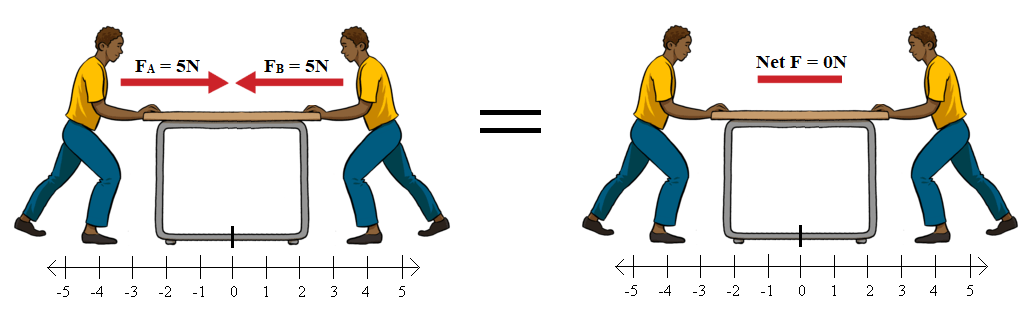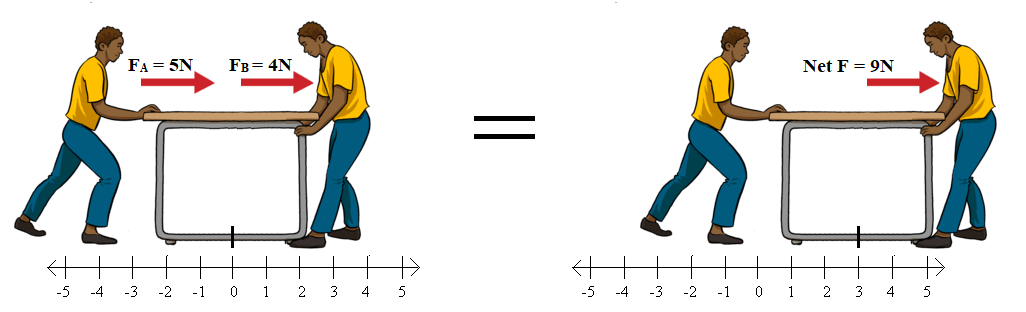# VOLTAGE AND CURRENT

Voltage and current both are important aspects of an electrical circuit, that’s why one need to understand about these terms. Both of them are essentially required to drive an electrical circuit.

## VOLTAGE AND CURRENT CONCEPT

### CURRENT

Current is something that is flowing like water flowing in river is known as water current and for wind we say air current is high or low today, similar to these

Electric Current is the rate of flow of electric charge (electrons) in a closed circuit. Electric current will divide in parallel branches (different in different loops or mesh) of a circuit while remaining the same in one loop (mesh) of a circuit. Current also follows a low resistive path.

The symbol for current is the letter “I”. The SI (Système international) unit is the Ampere, denoted by “A”. In simple words charge particles (electrons) flow in a direction in a closed circuit which causes electric current in a circuit.

NOTE: It is interesting to know Electrons flow from the negative terminal to the positive. Conventionally, Current flows from the positive terminal to the negative.

### VOLTAGE

Voltage is also called an electromotive force. It is actually the potential difference of charges across two points/nodes/terminals in an electrical circuit. Voltage will drop in series resistance while the same in parallel branches of resistance, what we measure is actually voltage drop across load.

Voltage is present in an open circuit, that’s why when we insert line tester in plug but didn’t touch behind, it will not lead the LED glow but as we touched it, LED glows up and shows that line is active.

Voltage is denoted by the letter “V”. The standard unit of voltage is the Volt, also denoted by “V”.

To make it simpler take an example

1. We have a table and two person pushing it in opposite direction with same forces, for example “FA=5 N& FB=5 N” so net potential difference (FA – FB) is “0 N” so the table don’t move in any direction, where N is Newton unit of force2. We have a table and two person pushing it in opposite direction with different forces, for example “FA=7 N& FB=5 N” so net potential difference (FA – FB) is “2 N” so the table will move in one direction (because A have more force, table will move in direction in which A is pushing/pulling), where N is Newton unit of force3. We have a table and one person pushing and one person is pulling it in same direction with some forces(either same or different forces),for example “FA=5 N & FB=4 N” so net potential difference (FA + FB) is “9 N” so the table will move in direction of pushing/pulling with force of “9 N”, where N is Newton unit of forceConclusion: More the potential difference faster the table (electrons) move.

NOTE: To drive a circuit both current and Voltage are essentially required.

#### COMMON RELATION BETWEEN VOLTAGE AND CURRENT

V = IR

This formula is derived by German physicist Georg Ohm

Ohm’s law states that the current through a conductor between two points is directly proportional to the voltage across the two points. Introducing the constant of proportionality, the resistance, one arrives at the usual mathematical equation that describes this relationship

I V

I = V / R

Where I is the current through the conductor in units of amperesV is the voltage measured across the conductor in units of volts, and R is the resistance of the conductor in units of ohms. Ohm’s law states that the R in this relation is constant, independent of the current.

As

R = V / I

If current is 0 it makes resistance infinite, means in open circuit (current = 0) resistance is infinite no matter how much voltage is present.

## HOW ELECTRONS FLOW IN A CIRCUIT

First let us remember the basic concept of a magnet we all know, magnet has two poles one is the North Pole and other is the South Pole. “Like (same) magnetic pole” repel each other and “unlike (not same) magnetic poles” attract each other i.e. “North & South” (opposite) attract each other while “North & North or South & South” repel each other.Same the concept above, electrons are negatively charged and many electrons push one electron (because same charge repel each other) then another electron then another electron this chain continues and electron emits from the source come back to the source if circuit is closed this process cause to flow current (electric charge) in a circuit.

Electrons flow from the negative terminal to the positive. Current flows from the positive terminal to the negative (conventionally).

NEXT POST
HOW ELECTRICITY IS GENERATED

PREVIOUS POST
DIFFERENCE BETWEEN STATIC AND CURRENT ELECTRICITY

Subscribe
Notify of